# Solid Rectangular Section Civil Engineering (CE) Notes | EduRev

## Civil Engineering (CE) : Solid Rectangular Section Civil Engineering (CE) Notes | EduRev

The document Solid Rectangular Section Civil Engineering (CE) Notes | EduRev is a part of the Civil Engineering (CE) Course Advanced Solid Mechanics - Notes, Videos, MCQs & PPTs.
All you need of Civil Engineering (CE) at this link: Civil Engineering (CE)

Solid Rectangular Section

Next, we assume that the bar has a rectangular cross section of width 2b and depth 2h, as shown in ﬁgure 9.15. For this section the boundary is deﬁned by the function,

f(x,y) = (b2−x2)(h2 −y2).               9.72)

It can be veriﬁed that choosing the Prandtl stress function, φ as Cf(x,y) would not satisfy the governing equation (9.47). Hence, we assume a stress function of the form,

φ = µΩ[V (x,y) + b2 −x2],                 (9.73)

where V is a function of (x,y).
Substituting equation (9.73) in equation (9.47) and simplifying we obtain,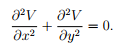Then, the boundary condition (9.50) requires that

V (±b,y) = 0, V (x,±h) = x2 −b2.            (9.75)

Thus, we need to solve the diﬀerential equation (9.74) subject to the boundary condition (9.75).

Towards this, we seek solution of the form

V = Vx(x)Vy(y),                        (9.76)

where Vx is a function of x and Vy is a function of y alone. Substituting the assumed special form for V , (9.76) in equation (9.74) and simplifying we obtain,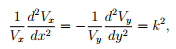(9.77)
where k is any constant. Recognize that the left hand side of the equation is a function of x alone and the right hand side is a function of y alone. Hence, for these functions to be same, they have to be a constant. Solving the second order ordinary diﬀerential equations (9.77) we obtain,

Vx = Acos(kx) + B sin(kx), Vy = C cosh(ky) + Dsinh(ky).                                                       (9.78)

Consistent with the boundary condition (9.75), we expect the functions Vx and Vy to be even functions, i.e., Vx(−x) = Vx(x) and Vy(−y) = Vy(y). Hence,

B = D = 0.                                                                                                         (9.79)

Substituting (9.79) in the equation (9.78) and the result into equation (9.76) we obtain,

V = AC cos(kx)cosh(ky) = E cos(kx)cosh(ky),               (9.80)

where E is some arbitrary constant. The equation (9.80) on applying the boundary condition (9.75a), i.e., V (±b,y) = 0 yields that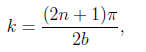(9.81)

where n is any integer. Since, the governing equation for V , (9.74) is a Laplace equation, it is a linear equation. Consequently, if two functions satisfy the given equation (9.74) then a linear combination of these functions also satisﬁes the Laplace equation. So the solution (9.80) can be written as,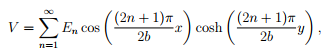(9.82)

where En’s are constant. Substituting (9.82) in the boundary condition (9.75b), i.e., V (x,±h) = x2 −h2 we obtain,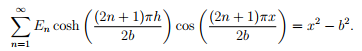(9.83)

Now, we have to ﬁnd the constants En such that for −b ≤ x ≤ b, a linear combination of cosines approximates the quadratic function on the right hand side of equation (9.83). Using the standard techniques in fourier analysis we obtain,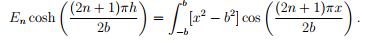(9.84)

ntegrating the above equation and rearranging, we obtain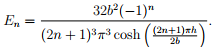(9.85)

Substituting equation (9.85) in (9.82) and the resulting equation in (9.73), we obtain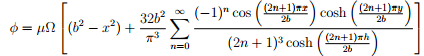( (9.86)

Having found the stress function, we are now in a position to ﬁnd the shear stresses and the warping function. First, we compute the stresses as,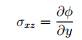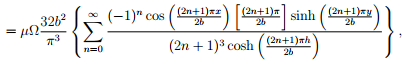(9.87)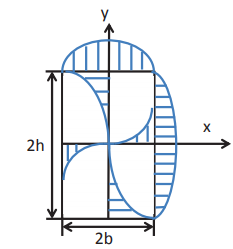Figure 9.16: Schematic of shear stress distribution in a bar with solid rectangular cross section subjected to end torsion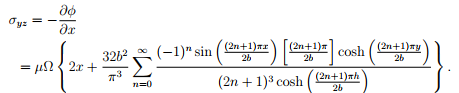(9.88)

Figure 9.16 schematically shows the shear stress distribution in a rectangular cross section along the sides of the rectangle and along the lines x = 0 and y = 0. The shear stress is maximum on the boundary and zero at the center of the rectangle. The maximum shear stress occurs at the middle of the long side and is given by,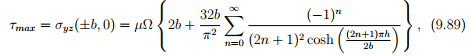where we have assumed that h ≥ b.

Substituting (9.87) in equation (9.45) and solving the first order ordinary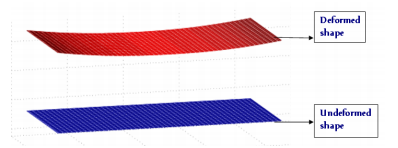Figure 9.17: Warping deformation of a rectangular section due to end torsion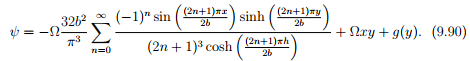Substituting equations (9.88) and (9.90) in equation (9.46) and solving the ﬁrst order ordinary diﬀerential equation for g we obtain g(y) = D0, a constant. Since, the bar does not move as a rigid body, we want ψ(0,0) = 0. Hence, D0 = 0. Consequently, the warping function is,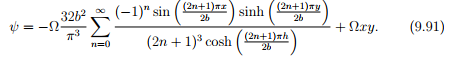Figure 9.17 plots the warped rectangular section. Substituting (9.87) and (9.88) in equation (9.57) we can relate the applied torque, T to the realized angle of twist per unit length, Ω as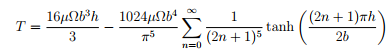(9.92)

This relation is expressed as

T = µΩC1(2h)(2b)3                            (9.93)

where C1 is a non-dimensional parameter which depends on h/b. In the same spirit, the maximum shear stress, (9.89) could be related to the applied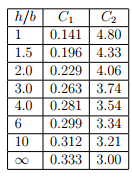Table 9.1: Tabulation of parameters C1 and C2 as a function of h=b

torque as,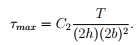(9.94)

where C2 is another non-dimensional parameter which depends on h/b. Values of C1 and C2 for various h/b ratios are tabulated in table 9.1. Thus, for thin rectangular sections, when h/b tends to ∞,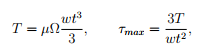(9.95)

where we have assumed the section to have a thickness, t = 2b and depth, w = 2h. It should be mentioned that irrespective of the orientation of the thin rectangular section, the equation (9.95) remains the same. This is because of the requirement that h ≥ b. Physically, irrespective of the orientation of the section, the torsion is resisted by the spatial variation of the shear stresses through the thickness of the section as shown in ﬁgure 9.19.

Offer running on EduRev: Apply code STAYHOME200 to get INR 200 off on our premium plan EduRev Infinity!

## Advanced Solid Mechanics - Notes, Videos, MCQs & PPTs

42 videos|61 docs

,

,

,

,

,

,

,

,

,

,

,

,

,

,

,

,

,

,

,

,

,

;